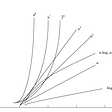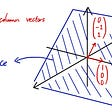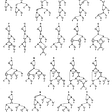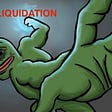# Turbocharging Derivatives — Variance, Convexity, and Everything in Between

My career in quantitative finance started about 15 years ago at a Tel Aviv-based fintech company called SuperDerivatives (now part of ICE). Their signature product suite was a user-friendly derivatives pricing library that could price anything from vanilla products to ultra-complexed exotic structures. My role there was a support representative, and given that back then I knew pretty much nothing about derivatives and quant finance, this was definitely a challenging role. As most of my interaction was with derivatives professionals, I literally had to learn a whole new language… most times, a normal conversation would be something in the likes of “Why is my <random asset> 6-wks, 30Δ put vol is priced <0.x> vol points off the interbank market?” or “why is my <Vanna/smile delta/any 3rd order greeks you can think of..> is off priced compared to my trusted excel spreadsheet???”

What I realized, after spending a few months on the support desk, is that in quant finance people talk in volatility and greeks (greeks as in derivatives, not the actual Greek language).

Now, most options traders that you speak with will talk in volatility terms, but the matter of fact is that they think in variance terms… You probably think it is essentially the same (because vol is just the square root of the variance, right?), but there is quite a difference between the two.

In this write-up, we will dive into the essence of variance/volatility and convexity and how their characteristics affect options pricing and modeling. After we understand the different features, we will go through the process of creating an extremely convex derivative product from scratch to illustrate how they affect the risk of such products.

So let our journey begin…

# The roots of convexity and Jensen’s Inequality

If you were to ask any options trader what they like about it, you would probably be told that it’s the convexity that makes options (and any non-linear product) so interesting. Convexity is the “juice” that makes options (and other non-linear products) far more attractive than owning the underlying asset (which is why one would be willing to pay/demand more for buying/selling the derivative product). While most believe that convexity only exists in non-linear products, the fact is that any derivative product (futures/forwards included) has some sort of convexity embedded in it (as its future path is drawn from a random sample). Before we go knee-deep into the practical applications of convexity in derivatives trading, let’s first understand what convexity is…

A function is considered to be convex if the line segment between any two points on the graph of the function doesn’t lie below the graph (between the two points). If that definition was a bit overwhelming and confusing, it’s easier to visualize that with a simple function. Let’s examine the following functions: f(x) = x , g(x) = x², h(x) = x⁴

As we can see above, for any two points that we choose on the x-axis, our y value will be smaller than our linear function (y=x).

The entire concept of convexity is well explained using Jensen’s Inequality (named after Johan Jensen). Jensen’s Inequality is a vital part of understanding randomness, volatility, and convexity. Anyone who really wants to understand derivatives pricing and risk should, IMO, start with an understanding of the intuition behind Jensen’s Inequality. So does this inequality means?

According to Jensen’s Inequality, if x is a random variable and f(x) is a function of a random variable, then the following inequality must hold:

Or, in plain English, the expected value of the function f(x) is ALWAYS greater (or equals) to the function of the expected value of variable x. If you are still not fully convinced that this inequality holds, let’s review the following example:

Let’s assume we want to price a CALL option on index XYZ (index level=100) with a strike price (k) = 100, and the only information that we have at time=0 (i.e., today) is that the value at expiry could be one of the following: 80, 90, 100, 110, 120.

let’s also assume the following payout function:

f(x) = max(x-k,0) where k=strike price

Now, we can calculate both E[f(x)] and f[E(x)] and see if the inequality holds:

Well, we can obviously see that the average payout for a call option is greater than the payout of the average outcome at maturity; hence, it satisfies Jensen’s Inequality.

One of the key byproducts of this inequality is the “time value” of options. As we price options assuming random walk (Brownian motion, a sample of random increments), and as we see that under any condition the expected value of the payout function will be positive before expiry (given a large enough sample), the option will have a “time value” (mainly because we don’t assume that the likely outcome will be the AVERAGE outcome, and assume some convexity in the future price). If we want to visualize that, let’s see how the value of a call option behaves as we approach the expiry.

Generally speaking, we can say that the root of convexity in derivatives lies in the fact that their prices are derived using a stochastic process (i.e., x=random variable). If we take our go-to underlying process, which is mostly a Brownian motion, we can see that the convexity in price is essentially a function of the randomness (expressed by the volatility). To put it more clearly:

This means that the change in S (spot price) = expected value of S + high order term (or, random variable * volatility). The higher our volatility, the greater the effect of the “high order” term on the terminal value of S. To emphasize that, let’s take two cases:

1. σ (annualized volatility) = 10%
2. σ = 20%

As we can see, under σ=20% the variation in the terminal value of S is far greater than σ=10%, which, as a result, increases the time-value of options (as our value grows at a rate = σ*sqrt(dt) )

## So now we can spot two main sources of convexity in derivatives:

1. The payoff function (i.e., non-linear payoff)
2. The underlying process dynamic, which is governed by the variance and time (the greater either one of them, the higher the value of our derivatives)

The last source of convexity, which essentially combines both the non-linearity of derivatives products and the underlying process dynamic, is the risk factors (greeks) of the products. If we think about Delta and Gamma, we can clearly see that their non-linearity is a source of convexity. If you are not fully sold on this idea, let take the following example:

We buy a 1-week 3% OTM call option on stock ZYX (let’s assume vol=10%). Soon after we buy the far OTM call option (remember, this is 3% away from the market), the underlying stock moves 1% (because of a fat-finger buying of someone who wanted to buy stock XYZ). Let’s see how the price and delta (option price sensitivity to the underlying) react to this move:

So what actually happened here, you probably ask…

As the underlying price moved higher toward our strike, the expected price of our stock moved higher. In addition, given that option price reflects the likelihood of the option ending up ITM (under the assumption of normal distribution), the probability increased in a non-linear way (1% move more than tripled our option value in % terms). This change is a direct result of two very important options greeks — Delta and Gamma. Plotting both w.r.t to spot basically tells the story about how they have a huge impact on convexity in non-linear products (such as options).

Being that both Delta and Gamma are non-linear in their nature, it doesn’t take too much for them to “kick in” once the underlying moves and affect the value of the option. In other words, they act as the leverage of the option (compared to owning the underlying asset).

So far, we’ve seen how the payoff function on non-linear products and the characteristic of a random process create convexity. Now we are going to highlight another highly important feature of variance that’s in the core of derivatives pricing — Additivity.

While we mostly measure (and talk) volatility, the correct way to measure the deviation in financial assets is using variance( vol²), and while it seems like a rather insignificant modification, it’s far from being insignificant.

The reason we are accustomed to measuring in terms of volatility is two-fold IMO:

1. Black-Scholes options pricing formula expresses the fluctuation parameter in terms of annualized standard deviation (volatility)
2. It’s easier for us to convert daily returns of asset prices to annualized volatility (i.e., 1% move to 16% vol using the “rule-of-16”), as everything grows at the rate of sqrt(t)

To understand why we should generally think in variance terms, let’s go back for a moment to middle-school math lessons and remember the basics of “Algebra of random variables.”

When combining two distributions (in our case adding), we need to use the variance of the sample the following way:

If we assume that the two distributions are independent of each other, we can re-write the sum of the two distributions as Var[X+Y]= Var[X]+Var[Y]

This, unfortunately, cannot be done on the standard deviation (volatility), which is why variance is the right way we should measure deviation and operate in the derivatives space.

## Let’s look at three widely used use-cases for variance in derivatives trading:

One of the most widely used trading strategies in volatility space is Forward-Volatility trading (FVAs in FX space, VIX futures in equity space). Forward vol strategy essentially takes a view on the level of a forward-starting volatility (either via FVA, which expires into a vanilla option, or VIX future that expires into cash). Let’s look at how the forward volatility is calculated:

Where :

T2 = back-end time to expiry , T1 = front-end time to expiry, t=value date (0), K=strike (either ATM or in Δ term)

As we can see, the forward vol between expires T1, T2 is derived from the weighted average variance (and then square-rooted to get the volatility). This could not be done if we were to use the volatilities instead.

Let’s look at the following volatility term structure to get a better understanding of pricing a typical FVA.

Let’s say we want to price a forward-starting 2-month ATM straddle (starting in 1-month). Given that we know 3-month vol= 17.6% and 1-month vol = 17.3%, we can derive the forward 1x3 FVA :

FVA(1x3) = sqrt[(17.6²*90–17.3²*30)/(60)] = ~17.75

2. Variance Swap Pricing

Variance Swap is an amazing product IMO, and while it has everything to do with convexity, the reason I’m mentioning it is that VarSwap is a perfect example of why variance is far easier to work with than volatility. Let’s start with a basic introduction to what VarSwap is(although I think most readers already know it by heart).

VarSwap is a forward contract that pays the difference between the realized variance of an asset (Say S&P500) and a pre-determined strike. If we want to mark-to-market our VarSwap position, it’s a relatively simple formula:

So as we can see the fact that variance (unlike vol) is additive in time, we can compute our VarSwap value as a weighted average between realized and implied variance. Furthermore, because of its time additivity, we can unwind opened VarSwap trades without accounting for realized fixings (something that cannot be done with the equivalent VolSwap). In essence, offsetting VarSwap risk is very similar to a vanilla option in its behavior, which makes it a superior product. To demonstrate the difference between VarSwap and VolSwap let’s take the following example:

We initiate two 1-month contracts (both long), VarSwap and VolSwap, at the same strike (obviously impossible, but let’s assume that we have a flat vol smile…). So we go long both swaps at a rate of σ=16%. For the first nine days (out of 20), the market doesn’t move whatsoever (daily returns are 0), so we decide to stop on our trade and sell 11days OUTRIGHT swaps for the remaining vega amount and the same vol strike (for some reason vol remained the same).

Due to our very bad luck, the day after we sold our swaps, the market started moving 2%/day for the next 11 days, let see the overall performance of our swaps

As we can see, selling outright VarSwap closed our exposure completely, while selling VolSwap exposed us further (i.e., not offsetting our risk at all). This happened for the exact reason of additivity (which is what differentiates between variance and volatility)

A lot can be said and written about correlation and the benefits of diversification in portfolio construction (MPT, APT, and CAPM to name a few), but in derivatives trading, like most cases, instead of using correlation as a parameter, we can trade it as an asset (very much like volatility). Products like Correlation Swap (FX), Dispersion Swap (Equities), and basket options essentially let traders trade implied/realized correlation the same way volatility is traded. Let’s look at how portfolio volatility (of two assets) is calculated:

Where Cov(i,j) = ρ(i,j) σ(i)σ(j)

As we can see, our portfolio volatility is a byproduct of the assets’ variance and the covariance, which means that we can isolate the covariance and essentially trade the correlation. In FX, volatility traders often use pair trading to take advantage of implied correlation mispricing. If we have liquidy traded options on FX currency pairs, we can easily back the implied correlation from them the following way :

Now you probably say, “That’s cool and everything, but how does it relate to convexity?”. If we look at the relationship between the three variances and correlation, we can see a non-linear relationship, which means that the sensitivity of our implied correlation to a change in one of the implied volatility levels will result in a convex move in correlation (think about it as leverage bet on implied volatility…). This notion can be extended to equities, where dispersion trades (either in the form of swaps or vanilla trades) are widely used to take advantage of correlation risk premium (mostly to sell historically overpriced correlation). Obviously, the topic of correlation trading can easily be discussed over a much longer write-up (which I will do sometime in the future…)

## Creating The Ultimate Convexity in Derivatives Space

So far we’ve seen how volatility/variance relate to convexity in derivatives space, and how using variance (instead of volatility) allows us to take advantage of the different aspects of volatility/correlation trading. Now, let’s try to create, from scratch, the ultimate product that offers an ocean of convexity…

The first step is to create an underlying asset with zero drift and about 20% vol (We will call XYZ stock). Because we want to enjoy the benefits of diversification, we will then bundle another 49 underlying assets (not necessarily stocks) that have the same volatility, but their average correlation is -0.2 into an index (we will call in not-SP50) and market that to CME-like exchange to launch futures on the index (and maybe find investment fund to create ETF later on). This index will have reduced volatility compared to its constituents, so pension funds and asset managers will love it.

Now that we have traded futures/ETF we can move on to start trading options on the futures (ideally a decent options chain that will have liquidity across a wide range of strikes and expires). The options chain will allow us to create a VIX-like volatility index (so some kind of 30-days variance swap vol index). As we cannot really trade the “spot” index, we will need to create a strip of futures (that act very much like FVA, or forward-forward volatility). Now, you are probably saying, “Yes…, but that’s really not enough convexity for me, my fund is looking to do x1000 returns, so we need more, can we have some extra leverage?”

This is where things can really go crazy…

So we have futures on forward-start VarSwap, that’s linked to options on future, that’s linked to an index, that’s…well, you know where this is going, right?

We can make it even more convex in payoff if we offer vanilla options on that future (so our underlying all of a sudden becomes to volatility future), or if we really wanna go crazy we can call our go-to french bank structuring desk and structure some weird note that’s contingent on the move in the realized correlation between the underlying index constituents...

Anyway, the point that I tried to make is that creating convexity from any random asset(s) is pretty easy if we understand the mechanism and how the leverage in derivatives products is generated.

I feel like I barely scratched the surface and there is much more that can be said and written about convexity and the infinite leverage that can be achieved as we go for higher-order exposures and derive our underlying further.

Harel.

--

--

--

## More from Harel Jacobson

Love podcasts or audiobooks? Learn on the go with our new app.

## Analysis of Algorithms## Linear Algebra 101 — Part 2## Solving the mystery behind Abstract Algorithm’s magical optimizations## Statistics: Hypothesis testing, power of the test## What is discrete mathematics??## The Perfect Matching: Part 1## Morphological Filters## More from Medium## Think Like a Market Maker — Understanding Implied Volatility## How to delta hedge an option: Part I## Atlas Algo Performance Update # 2